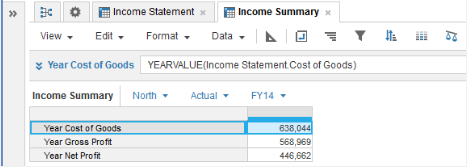1. Calculation functions
2. All Functions
3. Time and Date Functions
4. YEARVALUEReturns the year value of the source line item or property that matches the time summary method set for the source.

## Syntax

`YEARVALUE(x)`

where:

• x: Line item or property to be summarized.

## Format

Input Format Output Format

x: Line item - number, Boolean, date, time period, list, text.

Matches the input format.

## Arguments

The function uses the following arguments:

• x: Number, Boolean, date, time period, list item, text.

## Constraints

The function has the following constraints:

• The result formatting must match the source formatting.
• The result must have a timescale applied.
• If the time summary method is none, only numbers get values. Use other time summary methods to get values for Booleans, dates, time periods, list items, and text. See Summary Methods and Time Aggregation for more information.

## Excel equivalent

• No Excel equivalent

## Example

### Sum example

An Income Statement source module shows gross and net profit by region for Actual and Forecast versions: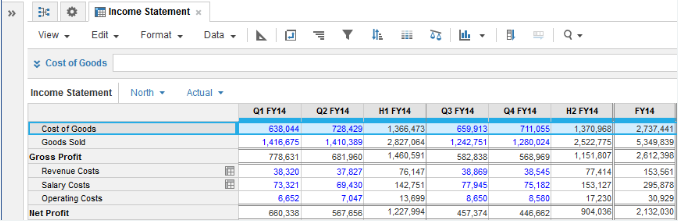For the Cost of Goods, Gross Profit, and Net Profit source line items, the time summary method is set to Sum: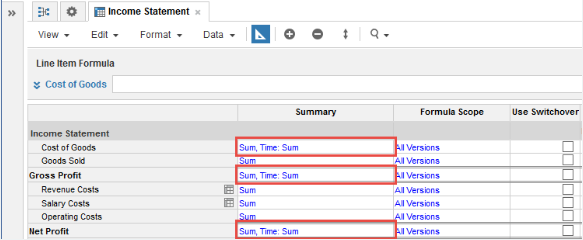You can use YEARVALUE in line items for an Income Summary module that has a Quarter timescale applied to pull in any required year totals:

`YEARVALUE(Income Statement.Cost of Goods)        `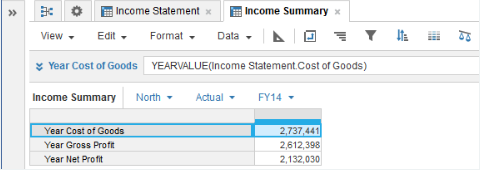Ensure that the time summary method for the result line items give you the values that you want. In this example, if you change the time summary method for the Year Cost of Goods result line item from Closing Balance to Sum, the value returned for the FY 14 selection, where the timescale for the result line item is set to Quarters, is incorrect.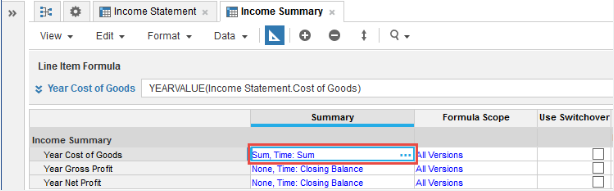The value returned is the multiple of each quarter's year value:
4 x 2,737,441 = 10,949,764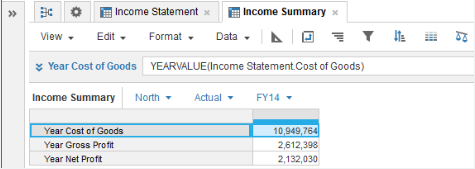### Min example

In this example, the time summary setting for the source line items is set to Min: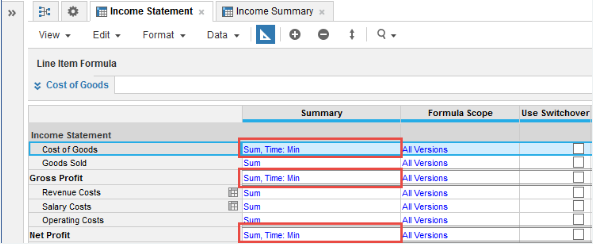The YEARVALUE result line items in the Income Summary module adjust to return the minimum quarter value for each year period:

`YEARVALUE(Income Statement.Cost of Goods)        `